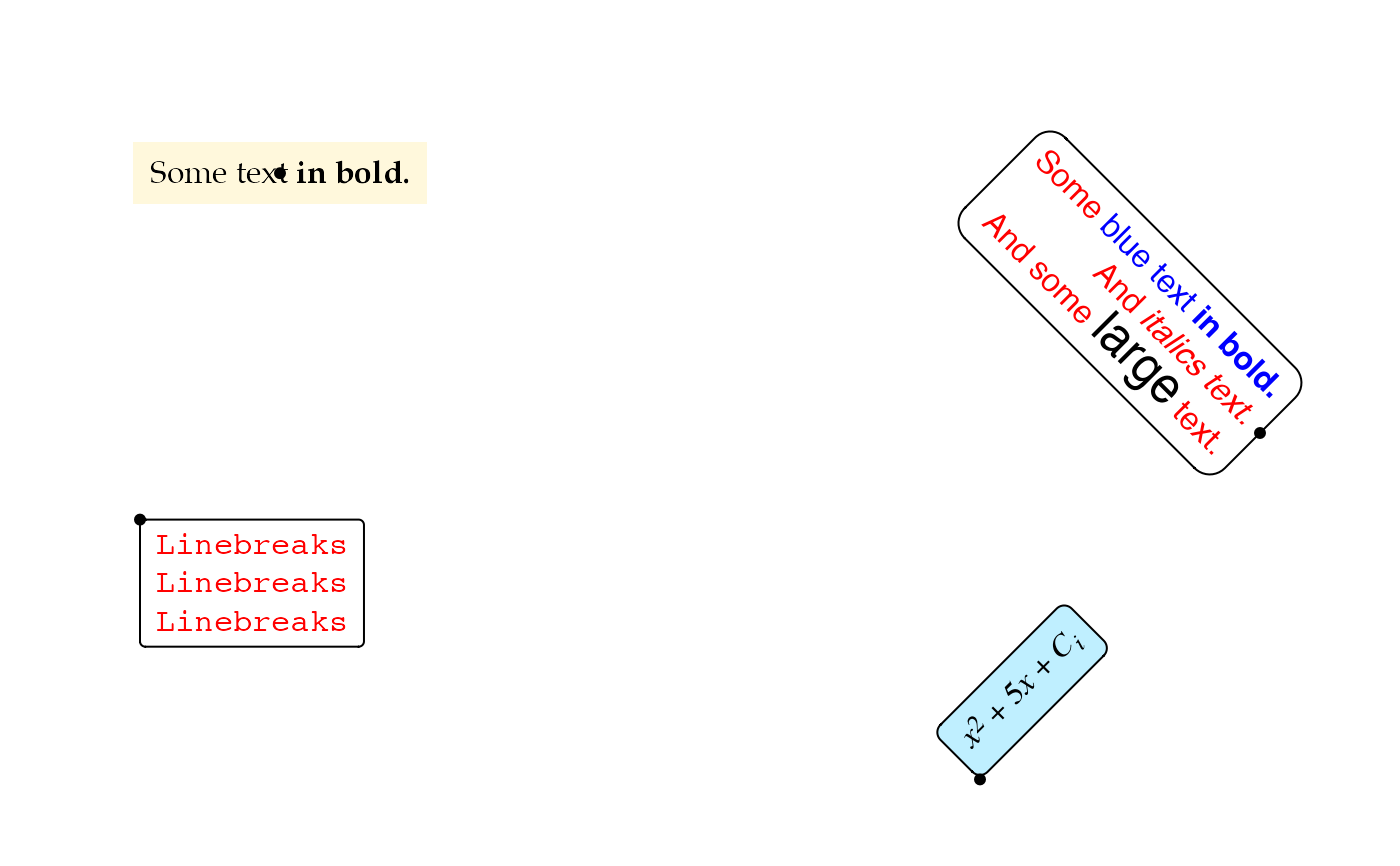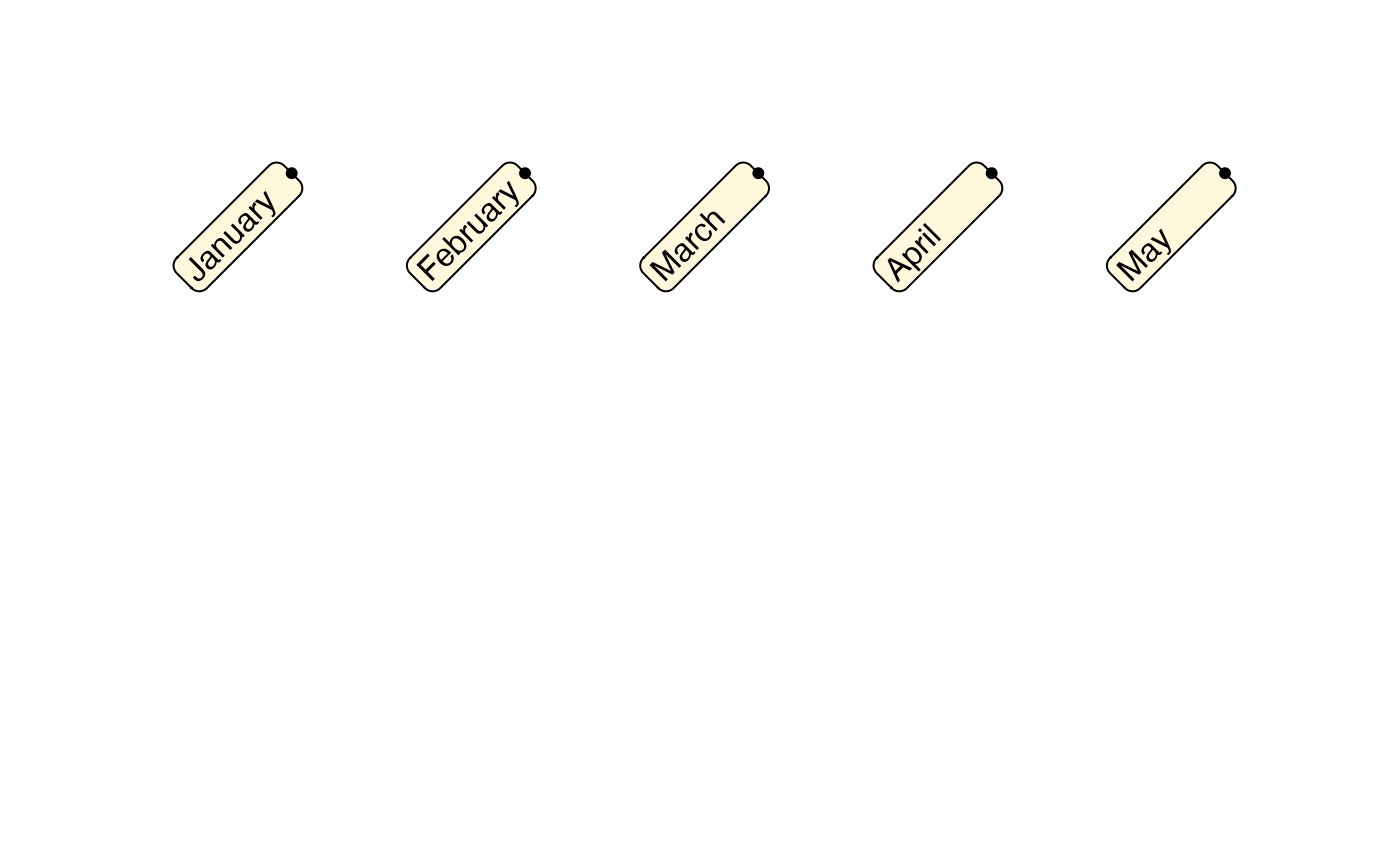This grob acts mostly as a drop-in replacement for grid::textGrob() but provides more sophisticated formatting. The grob can handle basic markdown and HTML formatting directives, and it can also draw boxes around each piece of text. Note that this grob does not draw plotmath expressions.

richtext_grob(
text,
x = unit(0.5, "npc"),
y = unit(0.5, "npc"),
hjust = 0.5,
vjust = 0.5,
halign = hjust,
valign = vjust,
rot = 0,
default.units = "npc",
margin = unit(c(0, 0, 0, 0), "pt"),
padding = unit(c(0, 0, 0, 0), "pt"),
r = unit(0, "pt"),
align_widths = FALSE,
align_heights = FALSE,
name = NULL,
gp = gpar(),
box_gp = gpar(col = NA),
vp = NULL,
use_markdown = TRUE,
debug = FALSE
)

## Arguments

text Character vector containing Markdown/HTML strings to draw. Unit objects specifying the location of the reference point. Numerical values specifying the justification of the text boxes relative to x and y. These justification parameters are specified in the internal reference frame of the text boxes, so that, for example, hjust adjusts the vertical justification when the text is rotated 90 degrees to the left or right. Numerical values specifying the text justification inside the text boxes. If not specified, these default to hjust and vjust. Angle of rotation for text, in degrees. Units of x and y if these are provided only as numerical values. Unit vectors of four elements each indicating the margin and padding around each text label in the order top, right, bottom, left. Margins are drawn outside the enclosing box (if any), and padding is drawn inside. To avoid rendering artifacts, it is best to specify these values in absolute units (such as points, mm, or inch) rather than in relative units (such as npc). The radius of the rounded corners. To avoid rendering artifacts, it is best to specify this in absolute units (such as points, mm, or inch) rather than in relative units (such as npc). Should the widths and heights of all the text boxes be aligned? Default is no. Name of the grob. Other graphical parameters for drawing. Graphical parameters for the enclosing box around each text label. Viewport. Should the text input be treated as markdown? Default is yes. Should debugging info be drawn? Default is no.

## Value

A grid grob that represents the formatted text.

textbox_grob()

## Examples

library(grid)

text <- c(
"Some text **in bold.**", "Linebreaks<br>Linebreaks<br>Linebreaks",
"*x*<sup>2</sup> + 5*x* + *C*<sub>*i*</sub>",
"Some <span style='color:blue'>blue text **in bold.**</span><br>And *italics text.*<br>
And some <span style='font-size:18pt; color:black'>large</span> text."
)

x <- c(.2, .1, .7, .9)
y <- c(.8, .4, .1, .5)
rot <- c(0, 0, 45, -45)
gp = gpar(col = c("black", "red"), fontfamily = c("Palatino", "Courier", "Times", "Helvetica"))
box_gp = gpar(col = "black", fill = c("cornsilk", NA, "lightblue1", NA), lty = c(0, 1, 1, 1))
hjust <- c(0.5, 0, 0, 1)
vjust <- c(0.5, 1, 0, 0.5)

g <- richtext_grob(
text, x, y, hjust = hjust, vjust = vjust, rot = rot,
padding = unit(c(6, 6, 4, 6), "pt"),
r = unit(c(0, 2, 4, 8), "pt"),
gp = gp, box_gp = box_gp
)
grid.newpage()
grid.draw(g)
grid.points(x, y, default.units = "npc", pch = 19, size = unit(5, "pt"))# multiple text labels with aligned boxes
text <- c("January", "February", "March", "April", "May")
x <- (1:5)/6 + 1/24
y <- rep(0.8, 5)
g <- richtext_grob(
text, x, y, halign = 0, hjust = 1,
rot = 45,
padding = unit(c(3, 6, 1, 3), "pt"),
r = unit(4, "pt"),
align_widths = TRUE,
box_gp = gpar(col = "black", fill = "cornsilk")
)
grid.newpage()
grid.draw(g)
grid.points(x, y, default.units = "npc", pch = 19, size = unit(5, "pt"))